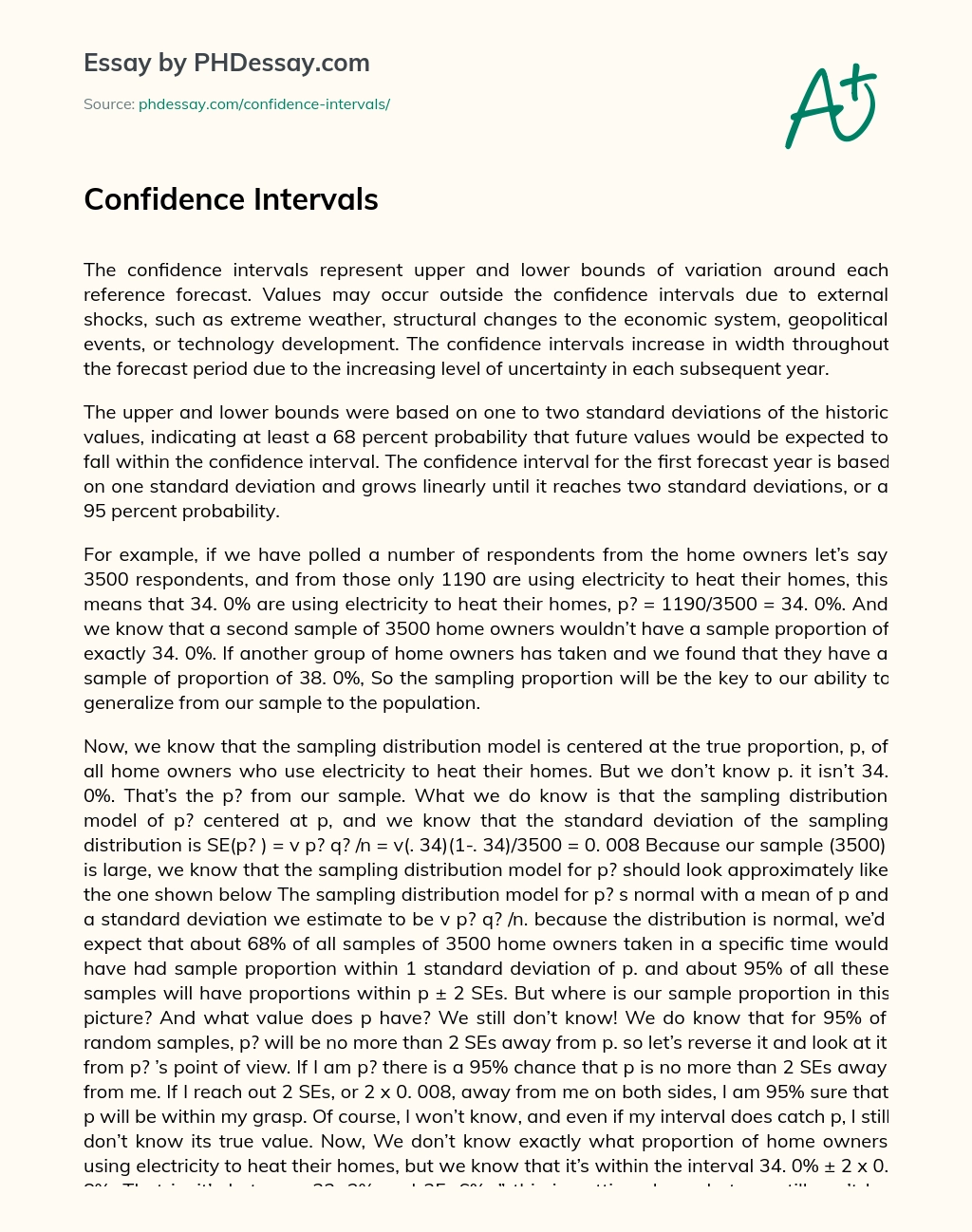Last Updated 27 Jan 2021

Confidence Intervals

Words 764 (3 pages)
Views 382

The confidence intervals represent upper and lower bounds of variation around each reference forecast. Values may occur outside the confidence intervals due to external shocks, such as extreme weather, structural changes to the economic system, geopolitical events, or technology development. The confidence intervals increase in width throughout the forecast period due to the increasing level of uncertainty in each subsequent year.

The upper and lower bounds were based on one to two standard deviations of the historic values, indicating at least a 68 percent probability that future values would be expected to fall within the confidence interval. The confidence interval for the first forecast year is based on one standard deviation and grows linearly until it reaches two standard deviations, or a 95 percent probability.

For example, if we have polled a number of respondents from the home owners let’s say 3500 respondents, and from those only 1190 are using electricity to heat their homes, this means that 34. 0% are using electricity to heat their homes, p? = 1190/3500 = 34. 0%. And we know that a second sample of 3500 home owners wouldn’t have a sample proportion of exactly 34. 0%. If another group of home owners has taken and we found that they have a sample of proportion of 38. 0%, So the sampling proportion will be the key to our ability to generalize from our sample to the population.

Order custom essay Confidence Intervals with free plagiarism report

GET ORIGINAL PAPER

Now, we know that the sampling distribution model is centered at the true proportion, p, of all home owners who use electricity to heat their homes. But we don’t know p. it isn’t 34. 0%. That’s the p? from our sample. What we do know is that the sampling distribution model of p? centered at p, and we know that the standard deviation of the sampling distribution is SE(p? ) = v p? q? /n = v(. 34)(1-. 34)/3500 = 0. 008 Because our sample (3500) is large, we know that the sampling distribution model for p? should look approximately like the one shown below The sampling distribution model for p? s normal with a mean of p and a standard deviation we estimate to be v p? q? /n. because the distribution is normal, we’d expect that about 68% of all samples of 3500 home owners taken in a specific time would have had sample proportion within 1 standard deviation of p. and about 95% of all these samples will have proportions within p ± 2 SEs. But where is our sample proportion in this picture? And what value does p have? We still don’t know! We do know that for 95% of random samples, p? will be no more than 2 SEs away from p. so let’s reverse it and look at it from p? ’s point of view. If I am p? there is a 95% chance that p is no more than 2 SEs away from me. If I reach out 2 SEs, or 2 x 0. 008, away from me on both sides, I am 95% sure that p will be within my grasp. Of course, I won’t know, and even if my interval does catch p, I still don’t know its true value. Now, We don’t know exactly what proportion of home owners using electricity to heat their homes, but we know that it’s within the interval 34. 0% ± 2 x 0. 8%. That is, it’s between 32. 2% and 35. 6%. ” this is getting closer, but we still can’t be certain. We can’t know for sure that the true proportion is in this interval-or in any particular range.

We don’t know the exactly the proportion of home owners that use electricity to heat their homes, but the interval from 32. 4% to 35. 6% probably contains the true proportion. ” We’ve now fudged twice—first by giving an interval and second by admitting that we only think the interval “probably” contains the true value. That last statement is true; we can tighten it up by quantifying what we mean by “probably. ” We saw that 95% of the time when we reach out 2 SEs from p? , we capture p, so we can be 95% confident that this is one of those times, after putting a number on the probability that this interval covers the true proportion,

We are 95% confident that between 32. 4% and 35. 6% of home owners using electricity to heat their homes, this is now an appropriate interpretation of our confidence intervals. It’s not perfect, but it’s about the best we can do. Level of Confidence = 95% =1- ? = 0. 05This essay was written by a fellow student. You can use it as an example when writing your own essay or use it as a source, but you need cite it.

Get professional help and free up your time for more important courses

Starting from 3 hours delivery 450+ experts on 30 subjects
get essay help 124  experts online

Did you know that we have over 70,000 essays on 3,000 topics in our database?